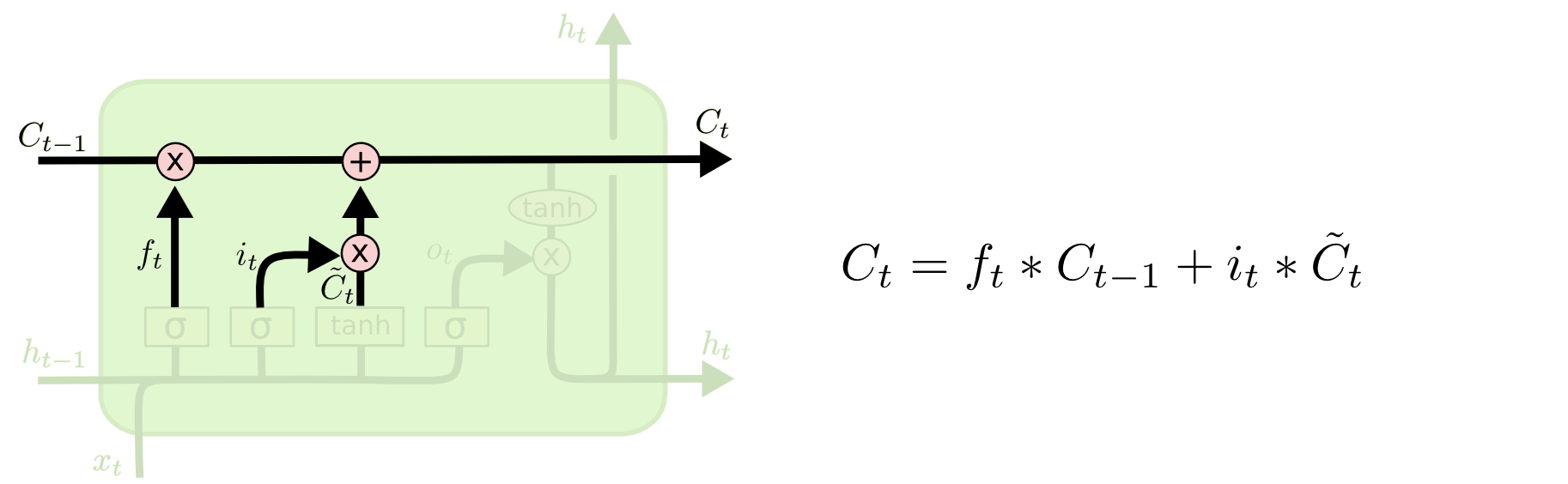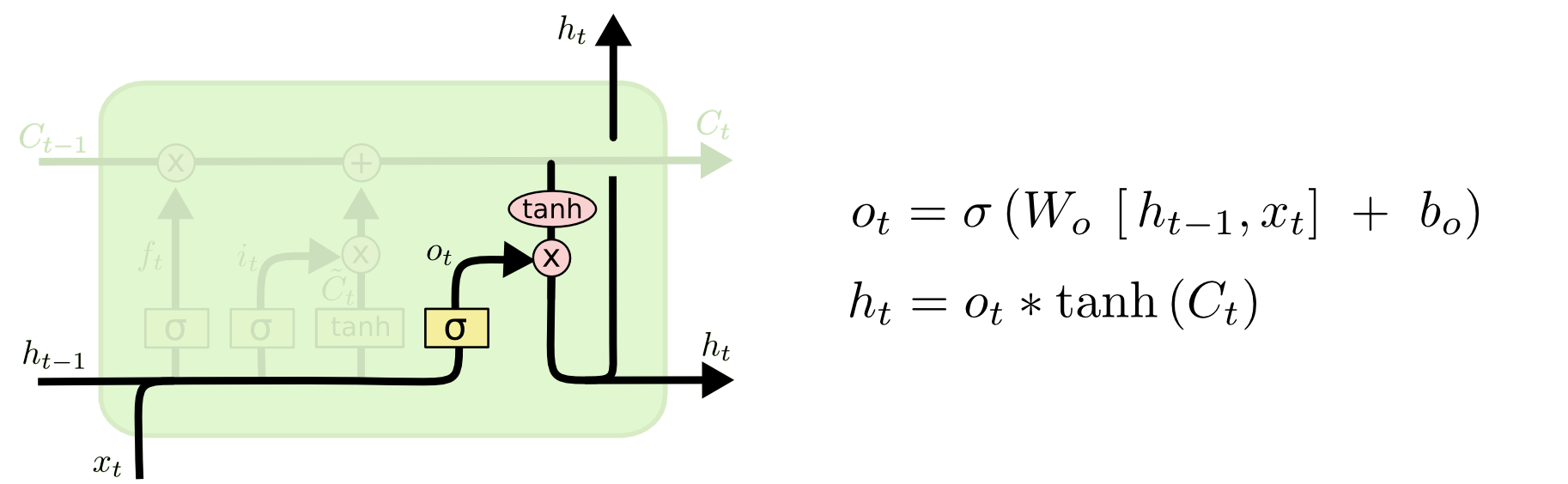## Keras 中 LSTM 层两个重要参数理解keras.layers.LSTM 类中有两个常用但不容易理解的参数，分别是 return_sequencesreturn_state。对于两个参数，官方定义如下：

• return_sequences: 布尔值。是返回输出序列中的最后一个输出，还是全部序列。
• return_state: 布尔值。除了输出之外是否返回最后一个状态。keras.layers.LSTM 默认 return_sequences=Falsereturn_state=False。此时只会返回最终 $h_t$ 值。例如：

# 第一次实验
import numpy as np
import tensorflow as tf

# 建立模型
inputs = tf.keras.layers.Input(shape=(3, 1))
lstm = tf.keras.layers.LSTM(1)(inputs)
model = tf.keras.models.Model(inputs=inputs, outputs=lstm)
# t1, t2, t3 序列
data = np.atleast_3d([0.1, 0.2, 0.3])
model.predict(data)

    array([[-0.07383346]], dtype=float32)

# 第二次实验
inputs = tf.keras.layers.Input(shape=(3, 1))
lstm = tf.keras.layers.LSTM(1, return_sequences=True)(inputs)
model = tf.keras.models.Model(inputs=inputs, outputs=lstm)

model.predict(data)

    array([[[0.00679269],
[0.01709353],
[0.02898963]]], dtype=float32)

# 第三次实验
inputs = tf.keras.layers.Input(shape=(3, 1))
lstm = tf.keras.layers.LSTM(1, return_state=True)(inputs)
model = tf.keras.models.Model(inputs=inputs, outputs=lstm)

model.predict(data)

    [array([[-0.01886126]], dtype=float32),
array([[-0.01886126]], dtype=float32),
array([[-0.04175678]], dtype=float32)]

# 第四次实验
inputs = tf.keras.layers.Input(shape=(3, 1))
lstm = tf.keras.layers.LSTM(
1, return_sequences=True, return_state=True)(inputs)
model = tf.keras.models.Model(inputs=inputs, outputs=lstm)

model.predict(data)

    [array([[[0.01515806],
[0.04311799],
[0.08097965]]], dtype=float32),
array([[0.08097965]], dtype=float32),
array([[0.15623903]], dtype=float32)]